Mobile QR Code1. (School of Electronics and Electrical Engineering, Lingnan Normal University, Zhanjiang, 524048, China )

Intelligent image, SIFT algorithm, Gabor feature, CNN, Multi-source information, Feature extraction

## 1. Introduction

In the era of intelligence, people’s lives have become more convenient because of the rapid development of science and technology, and technologies in various fields are constantly developing. Many of these developments are inseparable from the intelligent monitoring of multi-source information images, and the intelligent monitoring of images is an essential part . Environmental radar monitoring, small-scale technology development, industrial manufacturing process, and a variety of medical-related analyses are inseparable from information image intelligent monitoring (Ed note: Compound adjectives that modify a noun are typically hyphenated but not when the first word of the adjective is an adverb ending with -ly.'') . In the continuous development of the current intelligent technology, multi-source information image monitoring needs to strive for accuracy and precision and achieve a certain efficiency to meet the current production needs . Many scholars have examined multi-source information image monitoring at this stage and applied or improved various models from various angles . Nevertheless, intelligent image information monitoring still has many problems, including low sensitivity and accuracy, requiring improvement and development. SIFT (scale-invariant feature transform) algorithms and CNNs (convolutional neural networks) are often used in image feature recognition, and Gabor filters are often used for the detection of various waveforms, including image signals. Therefore, the research is based on the Gabor features, using the SIFT algorithm, combined with feature extraction and the CNN enhancement of image recognition, to form a new improved algorithm, which is applied to multi-source information image monitoring.

## 2. Related Work

Image information monitoring has attracted considerable research attention. Weatheral et al. used near-infrared spectroscopy to build noninvasive monitors of human brains. The device includes three sensors that analyze the near-infrared spectrum to ensure adequate monitoring time. Such a device reduces the measurement time . Saunders et al. proposed a continuous monitoring and measurement method of equivalent radiation value based on the global numerical weather prediction model to obtain more accurate data records for climate monitoring. The rotation of visible and infrared imagers was used to enhance the stability of the monitoring model. The experimental results show that this method is more stable and less affected by noise . Using four different imaging techniques, Etienne et al. proposed a complete image analysis pipeline and extracted dynamic information on the seed imbibition process from such monitoring experiments. Different imaging methods were compared to determine the image monitoring method most suitable for the seed imbibition process . Scholars, such as Bekeneva, designed intelligent monitoring systems for power systems based on the Internet of Things by monitoring images to achieve dynamic supervision to improve the accuracy of computer room management, work efficiency and reduce the work intensity of computer room managers. The results showed that this method has significant advantages over traditional algorithms with better results .

Many studies have examined the SIFT algorithm, Gabor feature, and CNN. Chakrabarty et al. proposed a CNN-based supervised learning approach to estimate the direction of the arrival of multiple speakers. The experimental results showed that the proposed method could adapt to unknown acoustic conditions. It is robust to unknown noise types and can accurately locate speakers with different sound sources in dynamic acoustic scenes. . Feng et al. designed a sky image-based CNN model to predict the global horizontal irradiance one hour earlier using sky images without numerical measurements and additional features. The results showed its superiority in various weather conditions . Li et al. proposed a hybrid deep convolutional neural network model and applied it to solar flare predictions. The results revealed some breakthrough key features that the model can automatically extract and may provide important clues for examining the mechanism of flares . In studying the image classification of convolutional neural networks, Sun et al. used a genetic algorithm to design the automatic structure of a CNN. (Ed note: The use of it'' to begin a sentence should be avoided because it can sometimes create confusion as to what it'' is.)The algorithm could maintain the accurate classification of its images in a changing environment. They reported that this method effectively solves the image classification problem . Zhang et al. proposed an optical and synthetic aperture-radar-image-registration method based on OS-SIFT and cascaded sample consistency techniques to establish sufficiently reliable relationships between images. The accurate search space of the best matching points was constructed by combining the inherent characteristics of an optical image and a synthetic aperture radar image. The experimental results verify the robustness and accuracy of the proposed algorithm . Cordero et al. used the Gabor filter to analyze the Schrodinger-type evolution equation. The final result proved the accurate estimation of the Gabor matrix of the generalized type partial inverse operator. The improved Gabor has better sparsity, diffusion, and dispersion . Tian et al. designed a variety of decomposition functions and scale spaces based on the Gabor filter banks and descriptors to detect seismic waves more accurately. The simulation results showed that this method could improve the resolution of the wave rate .

Many studies have been performed on intelligent image information monitoring involving the SIFT algorithm. The Gabor feature and CNN can be well integrated into various fields, including image research. On the other hand, few studies combined the above methods to form new algorithms and analyze their performance. The research starts with the SIFT algorithm, Gabor feature, and CNN. An improved algorithm is constructed and applied to multi-source information image monitoring to obtain a better effect of intelligent monitoring of images.

## 2. Application of the SIFT Algorithm Combined with Gabor Feature and CNN in Multi-source Information Image Monitoring

### 2.1 Operation of the SIFT Algorithm in Multi-source Information Image Monitoring

Image registration is an important step in image monitoring, which performs geometric calibration on multiple images containing overlapping regions from different viewpoints in the same scene. This step is essential in multi-source information image monitoring. In current remote sensing image monitoring, it is usually automatic registration that requires the appropriate algorithms. The research uses the SIFT algorithm as the main remote-sensing image registration technology.

The SIFT algorithm is used mainly in general optical images with a small amount of additive Gaussian noise and related problems. Therefore, its design is also more inclined to general optical images, and its applicable image types are limited . In multi-source image monitoring, the template registration of the image domain is needed first. In the two images, the mutual information is maximized to solve the best matching model parameters between the images. (Ed note: Two complete sentences should not be combined with a comma.)

The entropy of image A is expressed as Eq. (1).

##### (1)
$H\left(A\right)=-\sum _{a}PA\left(a\right)\log PA\left(a\right)$

where $PA(a)$ represents the probability that a certain area in image A appears in the overall information. The entropy of image B is the same. The joint entropy of images A and B is shown in Eq. (2).

##### (2)
$H\left(A,B\right)=-\sum _{a,b}PAB\left(a,b\right)\log PAB\left(a,b\right)$

where $PAB(a,b)$ represents the probability that a certain area in the co-existing area of images A and B appears in the overall information. According to Eqs. (1) and (2), the information entropy of images A and B is expressed as Eq. (3).

##### (3)
$MI\left(A,B\right)=H\left(A\right)+H\left(B\right)-H\left(A,B\right)$

After obtaining the information entropy between the images, the phase correlation algorithm was used to perform Fourier transform, and the corresponding transform parameters were obtained. The translation is $(x_{0},y_{0})$ assuming that the scale of the image $f_{1}$ is zoomed $\alpha$. (Ed note: Short coordinating conjunctions like and'' and but'' should not be used at the beginning of sentences.) The linearly transformed image $f_{2}$ is obtained after the rotation angle $\theta$. Eq. (4) expresses the relationship between $f_{1}$and $f_{2}$, the sum $F_{2}$ obtained by Fourier transform in polar coordinates $F_{1}$.

##### (4)
$F_{2}\left(\rho ,\theta \right)=e^{\left(\rho {x_{0}}\cos \theta +\rho {y_{0}}\sin \theta \right)}\alpha ^{-2}F_{1}\left(\frac{\rho }{\alpha },\theta \right)$

where $\rho$ is the rotation angle corresponding to the peak position after the inverse Fourier transform. Different feature extractions are performed using the SIFT algorithm, among which the first is point feature extraction. Point feature extraction relies only on local information and uses some image elements to replace the entire image. In online feature extraction, the edge detection algorithm is used to extract the line features, and the matching lines are then matched according to the feature descriptors. Face feature extraction is performed after the line features are obtained. The surface feature is mainly to extract the closed area of the image and perform a feature calculation according to the closed area, and use the feature as a description of a certain area. The surface features often identify large areas, such as large waters, cities, forests, and deserts. The multispectral images of these areas have different spectral components, which are easy to identify and monitor. The virtual structure feature is finally extracted after the surface feature extraction is completed. The virtual structure is obtained using the above three basic actual structure features, and the corresponding virtual features were obtained by matching according to the similarity criterion . Fig. 1 shows the basic process of image registration of the SIFT algorithm.

In crucial point detection, the Gaussian scale difference space is generated using the Gaussian function, and the scale space of the image $S(x,y,z)$ is the convolution of the Gaussian function with the scale parameter and the image $s(x,y)$, as shown in Eq. (5).

##### (5)
$S\left(x,y,z\right)=G\left(x,y,z\right)\ast s\left(x,y\right)$

where $\ast$is a convolution operator; $G(x,y,z)$is a two-dimensional Gaussian function, and its calculation is expressed as Eq. (6).

##### (6)
$G\left(x,y,z\right)=\frac{1}{2\pi z^{2}}e^{-\frac{x^{2}+y^{2}}{2z^{2}}}$

In the scale space, multiple groups form a group $z$, and the calculation of the response value image $D(x,y,z)$ is expressed as Eq. (7).

##### (7)
$D\left(x,y,z\right)=S\left(x,y,kz\right)-S\left(x,y,z\right)$

where $k$ is the space multiple constants of adjacent scales. Using the response value image can simplify the computation, and the SIFT algorithm also constructs an image pyramid through the response value to realize the operation . The pyramid is divided into groups $o$, each group is a layer $s$, and the images of each group are obtained by down-sampling the previous group of images. Each group of images whose adjacent height is the scale space is described in detail. The response value $D$is obtained, and a pyramid is constructed with two sets of Gaussian scale space images. Each group of pyramids has five layers of space images of different scales. After the images of two adjacent layers meet, four layers of $D$ response values are obtained, and the key points are detected on these four layers. The second group is obtained by reducing the scale space of the first group by two times the resolution, and the image area is 25% of the previous group. Fig. 2 presents the scale pyramid.

At this time, in SIFT, the relationship between $z$and $o$ $s$is expressed as Eq. (8).

##### (8)
$\omega \left(o,s\right)=\sigma ^{{2^{o+\frac{s}{2}}}}$

where $\omega$is the number of groups; $\sigma$is the scale of the reference layer. After obtaining the critical point in the scale space that are invariant to image scaling and rotation, SIFT uses the principal direction and axis direction of each key point to generate descriptors and keeps these descriptors invariant as far as possible. The method completes the monitoring of the image.

##### Fig. 1. Basic flow of the SIFT algorithm registration.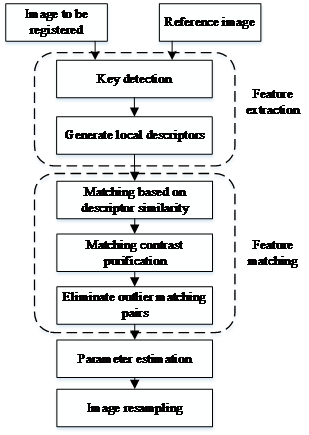##### Fig. 2. Schematic diagram of the scale space pyramid.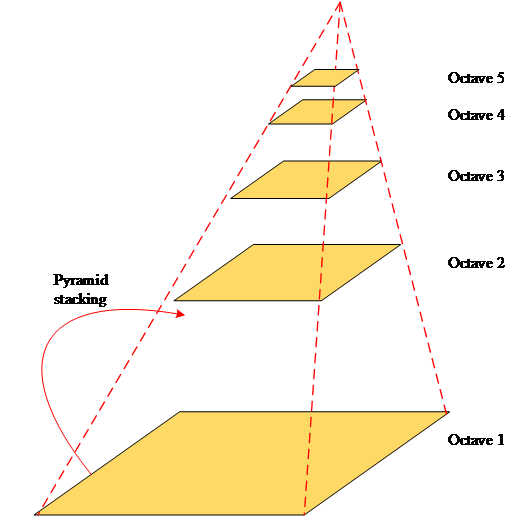### 2.2 Improvement of the SIFT Algorithm based on Gabor and CNN

Although the conventional SIFT algorithm can solve many image monitoring problems, the effect is not ideal on some specific images, such as synthetic aperture radar (SAR) images. In SAR images, the speckle noise effect of radar imaging cannot be avoided, and the signal-to-noise ratio of the matching feature space is also reduced significantly. The interior points are more difficult to filter out during feature matching. In addition, the Gabor filter has its advantages in detecting, and the CNN can simultaneously grasp local and global features when processing pictures. Therefore, combining a Gabor filter with CNN can achieve intelligent recognition of pictures. The research combines the Gabor descriptor and CNN with SIFT to form an improved algorithm to solve the monitoring problem of multi-source image information.

In the Gabor texture, the image does not depend on the reflection of color and brightness; it has high efficiency and homogeneity. In the spatial domain, the Gabor filter is usually regarded as a sine plane wave modulated by a Gaussian function, and its function representation is expressed as Eq. (9).

##### (9)
$f\theta ,\gamma ,\delta \left(x,y\right)=e^{-\frac{x^{2}+y^{2}}{2\delta }}\cdot e^{\frac{\pi }{\gamma \delta }\left(x\sin \theta -y\cos \theta \right)}$

where $\gamma$ is the frequency parameter; $\delta$ is the scale parameter; $\theta$is the direction parameter; $(x,y)$ is the pixel coordinate in the space domain. Any filter can be obtained by translation, rotation, or scale transformation through the Gabor filter. The Gabor kernel function is the waveform phase function. The Gabor kernel function $X$is convolved with the image, and the obtained Gabor response is expressed as Eq. (10).

##### (10)
$F\theta ,\gamma ,\delta =f\theta ,\gamma ,\delta \ast I$

The Gabor response is always complex, which is the extracted Gabor feature. The Gabor feature reflects some specific features of the image, including the edge direction information, texture direction information, and scale information of the image. The Gabor filter bank used in the study contains three scales and eight directions with 24 filters. The Gabor kernel function increases from the top to the bottom of the scale function, and the radian of each clockwise rotation from left to right is ${\pi}$ /8.

A 24-dimensional local Gabor feature descriptor is selected to reduce the computation time of the Gabor features as much as possible. With the support of the SIFT descriptor, the width of the support domain window is $m\cdot d\cdot \delta$; $m$ is the size parameter of the sub-region. The research is set to 3. The number of subregions $d$is the parameter, and the research is set to 4; $\delta$is the same as the previous one and is the key point scale parameter. To generate Gabor descriptors, Gabor filter banks are first generated, and images of specific regions required to generate the descriptors are then selected. After obtaining a specific corresponding area, the SIFT algorithm is used to calculate the relevant scale of the feature map, and the feature map of 33 ${\times}$ 33 pixels is obtained. Finally, a Gaussian weighted average is obtained, and the eigenvalue vector is the Gabor descriptor . After obtaining the Gabor descriptor, the feature vector needs to be normalized, as shown in Eq. (11).

##### (11)
$F_{Gabor}=\frac{F_{Gabor}}{\sqrt{\sum F_{Gabor}}}$

In Eq. (11), $F_{Gabor}$ is the descriptor before normalization and $F_{Gabor}$is the descriptor after normalization. For the key points $\delta$of each scale, the research uses two mutually nested support regions as the basis for generating descriptors to complete the fusion of descriptors. The two support regions are of different sizes, but both have an average of 24 dimensions.

As the basis of convolution operation, CNN exists as a basic operation in the SIFT and Gabor operations and strengthens the enhancement of the entire algorithm for multi-source-information image monitoring because of its sensitivity to image features. The components of CNN include the convolution operator, convolution feature kernel, convolution layer, and pooling layer. The structure is divided into an input layer, convolution layer, activation function, pooling layer, and fully connected layer. Fig. 3 presents its simple structure.

##### Fig. 3. Basic structure diagram of the CNN.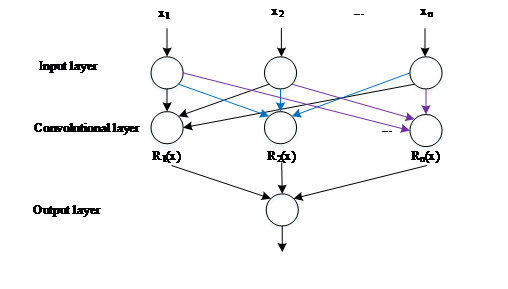##### Fig. 4. Schematic diagram of the pooling operation.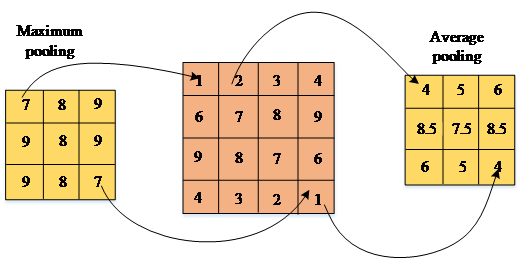The input layer is a pixel matrix, which performs various processing on the sample data, including data normalization, dimensionality reduction, pixel correction, and scale normalization. The convolutional layer contains multiple feature data. By learning the feature expression, the local area is processed by local perception, and the processed object is the corresponding feature data. A synthesis operation is then performed on each part, which integrates the information of each part, and obtains the global information through the convolution operation. The convolution process is relatively stable because the size of the convolution kernel weight does not change due to parameter sharing during the convolution process. The output of the convolutional layer is input to the activation layer. The activation function of the activation layer is operated. The activation function generally adopts the sigmoid function. In some cases, the Gaussian kernel function or spatial function can be used . The method of activation function operation is nonlinear mapping. At this time, the convolution layer can extract more abstract features and improve the function of the convolutional neural network. The sigmoid function is shown in Eq. (12).

##### (12)
$h_{\theta }\left(t\right)=\frac{1}{1+e^{-{\theta ^{t}}}}$

where $\theta$ is the mapping of the Sigmoid function. The first feature map $f_{k}$ obtained after the sigmoid operation $k$is expressed as Eq. (13).

##### (13)
$f_{k}=sigm\left(W^{k}x+b^{k}\right)$

where $x$is the input value; $W$is the weight; $b$is the bias. The pooling layer is between the two convolutional layers. The function of the pooling layer is to reduce the size of the parameter matrix and the overall number of relevant parameters of the fully connected layer. Pooling operations usually include max pooling and average pooling. Fig. 4 shows the method of pooling operations.

The pooling layer affects the parameters of the fully connected layer. The fully connected layer is generally at the end of the entire CNN structure and usually has several layers. The main function of the fully connected layer is to transform each local feature extracted by the convolutional layer into a whole through the weight operation to obtain a more complete and hierarchical overall feature. Assuming an input initial feature map of each convolutional layer $x_{j}$, the convolution operation is expressed as Eq. (14).

##### (14)
$x_{j}^{l}=f\left(\sum _{i\in M_{j}}x_{i}^{l-1}\cdot k_{ij}^{l}+c_{j}\right)$

where $f(x)$ is the activation function; $M_{j}$represents the set of initial feature maps; $i$ is the matching result; $k_{ij}$ is the input of the first feature map $i$ and the convolution kernel of the output of the first feature map$j$.

In order to solve the weights$l$ and update values of all neurons on the layer, it is necessary to find the sensitivity at each node first. The size $\theta$ is then calculated, and the corresponding parameters $l$ required by the layer through the sensitivity size are deduced. The sum of the sensitivity values from the connection layer $l$ to the interest definition $l+1$ is multiplied $\theta _{j}^{l+1}$by the corresponding weight $W$. The activation function is obtained using the reciprocal $f(u^{l})$to obtain Eq. (15).

##### (15)
$\theta _{j}^{l}=\theta _{j}^{l+1}W_{j}^{l+1}\cdot f\left(u^{l}\right)$

where $u$is the input value of the neurons of the layer $l$. A modified linear activation is applied to the CNN structure. The 1${\times}$1 convolution can reduce the dimension of the feature map to expand the application scale of the network, increase the width and depth of the convolutional neural network, and improve the application performance of the network. The operation process of CNN mainly strengthens the image features, which can theoretically shorten the operation time and increase the recognition accuracy. Integrate Gabor and CNN into the SIFT algorithm to form the Gabor-CNN-SIFT algorithm. Fig. 5 presents the flow of the entire algorithm.

The improved algorithm and other algorithms were simulated and compared. The samples of the simulation experiment were 240 different multi-source information images. In addition to the improved Gabor-CNN-SIFT algorithm, the other two algorithms were the Gabor-SIFT and only SIFT algorithms, of which Gabor-SIFT is an algorithm that does not combine CNN and only combines Gabor. The samples were randomly divided into two sets, including the test set and the validation set, to obtain the test results more accurately.

##### Fig. 5. Basic flow chart of the Gabor-CNN-SIFT algorithm.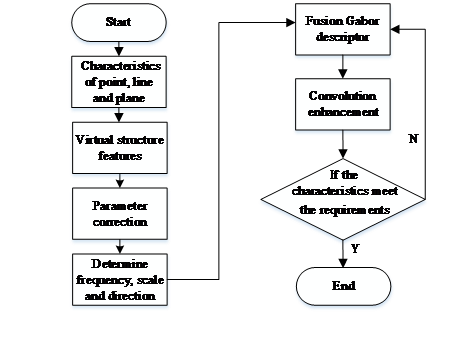## 3. Simulation Results and Analysis under the Comparison of Three Algorithms

The hardware environment of the performance test was an I7-8750 processor, 16G memory, and 2T hard disk, and the programming environment was PYTHON. The performance test of the algorithm mainly included the accuracy rate, precision rate, recall rate, ROC and PR curve, and the result value of the harmonic function F1 to reflect the comprehensive balance ability of the algorithm. The detection method compared the number of pixels between the simulation results of each method and the actual results. The study selected 5000 monitoring images in the ImageNet, and divided them into simple images and multi-scene images manually according to the complexity of the images. Owing to the poor monitoring effect of previous image monitoring methods on multi-scene images, this study selected 2832 multi-scene images as the data set for algorithm comparison.

The accuracy of the three algorithms when the iteration time was not short (Fig. 6). The accuracy of each algorithm showed a clear upward trend when the iteration time was increased. Among them, the accuracy of the Gabor-CNN-SIFT algorithm was higher than the other two algorithms in both the test set and validation set. In the average results obtained, the average accuracies of the three algorithms Gabor-CNN-SIFT, Gabor -SIFT, and SIFT are 92.35 %, 76.29%, and 79.23 %, respectively. Using significance analysis to judge the three average values, the average value of Gabor-CNN-SIFT was significant with the other three algorithms. Hence, the accuracy of the algorithm is significantly higher than the other two algorithms.

Fig. 7 shows the accuracy of each algorithm as the number of iterations increases. The curve of Gabor-CNN-SIFT in the three algorithms was always on the top (Fig. 7), indicating that the accuracy of this algorithm is always higher than the other two algorithms. In the average results obtained, the average accuracies of the three algorithms, Gabor-CNN-SIFT, Gabor-SIFT, and SIFT, were 82.55 %, 73.28 %, and 69.95 %, respectively. Using the significance analysis to judge the three average values, it was concluded that the average value of Gabor-CNN-SIFT was significant with the other three algorithms. Hence, the accuracy of the algorithm is significantly higher than the other two algorithms. The granularity of the overall verification of the example has a clear performance advantage.

##### Fig. 6. Accuracy rate of the three algorithms along with the iteration time.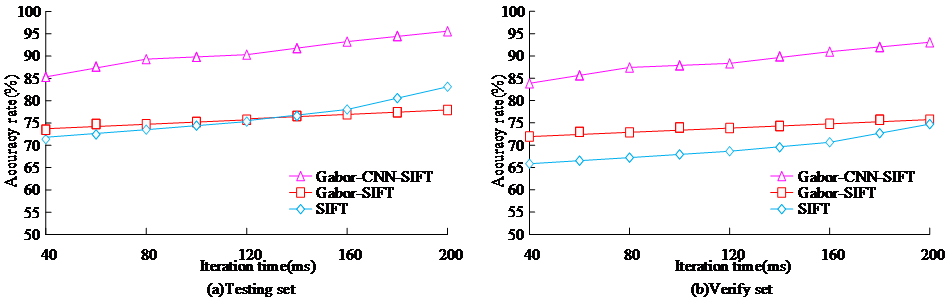##### Fig. 7. Precision rate results of the three algorithms with the iteration time.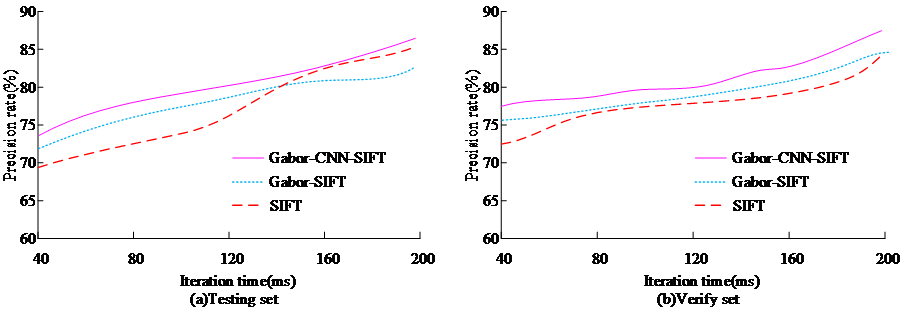##### Fig. 8. Recall rate results of the three algorithms with the iteration time.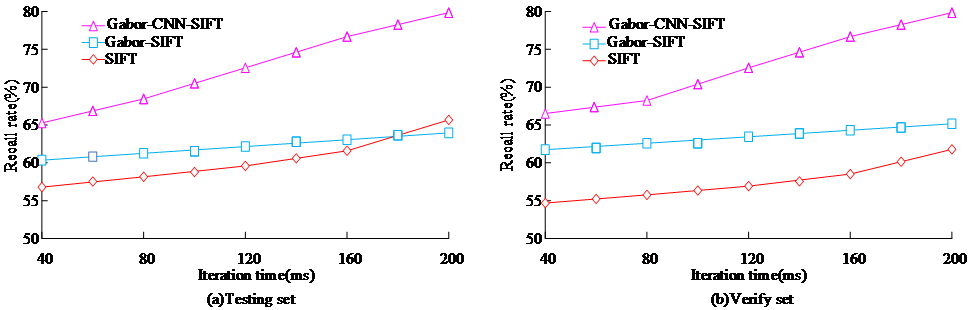##### Fig. 9. ROC curve and PR curve of the three algorithms.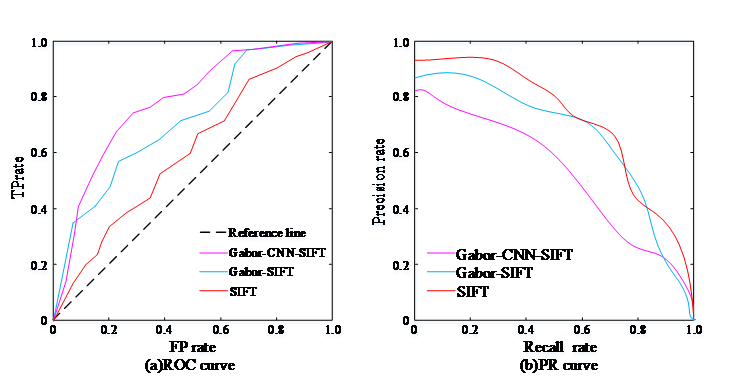In the simulation results, the recall rates of the three algorithms varied with the number of iterations, as shown in Fig. 8. The recall rate of each algorithm also showed an apparent upward trend when the number of iterations increased (Fig. 8). The recall rate of Gabor-CNN-SIFT was significantly higher than the other two algorithms in both the test and validation sets. Taking the average results, the average recall rates of the Gabor-CNN-SIFT, Gabor-SIFT, and SIFT algorithms were 74.79 %, 67.59%, and 66.92%, respectively. Using the significance analysis to judge the three average numerical results, it was concluded that the average value of Gabor-CNN-SIFT was significant compared to the other two algorithms. Therefore, the Gabor-CNN-SIFT is more conventional than the conventional algorithm. This algorithm has a significant performance advantage in the numerical judgment of negative examples.

##### Fig. 10. F1 score results of three algorithms along with the iteration time.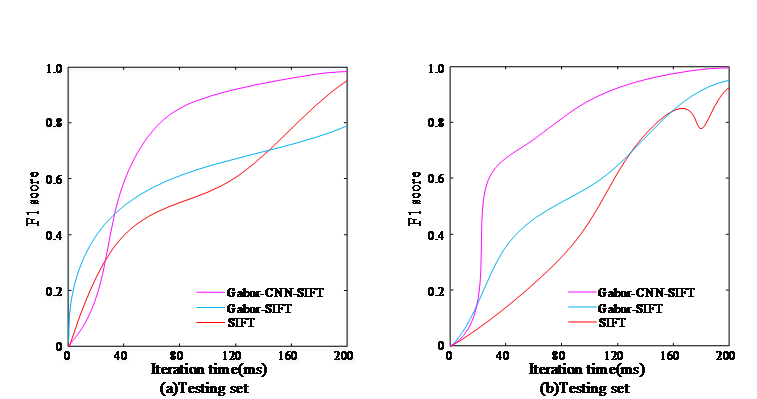Among the three algorithms, Fig. 9 shows the ROC curve and PR curve results of the comprehensive test set and validation set. The area under the ROC curve of the Gabor-CNN-SIFT algorithm was significantly higher than that of the other two algorithms (Fig. 9). The area under the ROC curve represents the comprehensive performance of the test accuracy of positive and negative examples. The area under the PR curve of the Gabor-CNN-SIFT algorithm was smaller than the other two algorithms, and the area under the PR curve represents the possible offset of the prediction. According to the calculated areas under the curve, the areas under the ROC curve of the Gabor-CNN-SIFT, Gabor-SIFT, and SIFT algorithms were 0.843, 0.741, and 0.592, respectively. The areas under the PR curve of the three algorithms were 0.466, 0.575, and 0.543, respectively. Combining the results of the ROC curve and PR curve, the Gabor-CNN-SIFT algorithm has a significant comprehensive performance advantage in the prediction and solution of data.

In the simulation results, the results of the three algorithms were synthesized. Fig. 10 shows the results of the obtained harmonic function F1 value changing with the number of iterations. The F1 score of the Gabor-CNN-SIFT algorithm in the test and validation sets was consistently above 80 and was significantly higher than the F1 score of the other two algorithms. The average results obtained F1 scores of the Gabor-CNN-SIFT, Gabor-SIFT, and SIFT were 0.89, 0.71, and 0.75, respectively. Significance analysis was used to compare the average results of the three algorithms, and it was concluded that the average F1 score of Gabor-CNN-SIFT was significantly different from the average F1 score of the other two algorithms. In balance, Gabor-CNN-SIFT has significant advantages over the other two algorithms.

## 4. Conclusion

This study examined ways to solve the problem of multi-source information image monitoring and ensure the normal operation of the intelligent monitoring of various images. The research combined the SIFT algorithm with Gabor features and CNN to form an improved Gabor-based feature enhancement based on the SIFT and Gabor descriptor features. The CNN-SIFT algorithm and the other two algorithms, including the Gabor-SIFT and SIFT, were tested and compared using the same data simultaneously. The simulation experiments showed that the average precision rate, average recall rate, average precision rate, and average F1 value of the improved Gabor-CNN-SIFT algorithm were 92.35 %, 74.79 %, 82.55 %, and 0.89, respectively. These results were significantly higher than the other algorithms and at a high level relative to the standard. The area under the curve of the ROC curve of the Gabor-CNN-SIFT algorithm was 0.843, which was larger than the other two algorithms. The area under the PR curve was 0.466, which is smaller than the other two algorithms. Hence, the performance of the improved algorithm was confirmed. The experimental results showed that the improved Gabor-CNN-SIFT algorithm has significant performance advantages in multiple performances and can be applied to multi-source information image monitoring. Although the research achieved certain results, the simulation experiments did not compare the calculation speed of the algorithms, and the improvement of the SIFT algorithm was also less. The improvement potential will also be a major direction of further research in the future.

### Fundings

The research is supported by: Natural Science Foundation of Guangdong Province for General Program in 2021 year, “Study on Hyperspectral monitoring mechanism and quantitative estimation model of nitrogen nutrition in seawater rice(2021A1515012440)” Lingnan Normal University Mangrove Institute for Key Program of Open Project in 2022 year, “Research on intelligent monitoring technology for mangrove in integrated air-ground(ZDXM02)”; National Natural Science Foundation for Youth Science Fund Project in 2021 year, “Research on mechanism and model of hyperspectral nondestructive testing and comprehensive performance evaluation of heavy metal pollution in shellfish(62005109)”; Guangdong Provincial Special Fund for Science and Technology Innovation Strategy (Key Project of the Climbing Plan)in 2022 year, “Integrated intelligent platform for deep sea fishery breeding based on Internet of things (pdjh2022a0312)”.

### REFERENCES

1
H. Chen, W. Li, & X. Xie. “Intelligent image monitoring technology of marine environmental pollution information”. Journal of Coastal Research 2020, 112(1), pp. 45-57.2
G. Li, Y. Ye, M. Zhou, et al. “Multi-resolution transmission image registration based on “Terrace Compression Method” and normalized mutual information”. Chemometrics and Intelligent Laboratory Systems 2022, 223, pp. 104-109.3
P. Chandra, D. Giri, F. Li, et al. “Advances in intelligent systems and computing information technology and applied mathematics,” Hamming Code 2019, 10, pp. 163-174.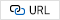4
R. Rodríguez, Y. Garcs, E. Torres, et al. “A vision from a physical point of view and the information theory on the image segmentation”, Journal of Intelligent & Fuzzy Systems, 2019 37, pp. 2835-2845.5
A. Weatherall, E. Poynter, A. Garner, et al. “Near‐infrared spectroscopy monitoring in a pre‐hospital trauma patient cohort: An analysis of successful signal collection”, Acta Anaesthesiologica Scandinavica, 2020, 64, pp. 117-123.6
R. W. Saunders, T. A. Blackmore, B. Candy, et al. “Ten Years of Satellite Infrared Radiance Monitoring With the Met Office NWP Model”, IEEE Transactions on Geoscience and Remote Sensing, 2021, 59, pp. 4561-4569.7
B. Etienne, D. Clément, G. Nicolas, et al. “Evaluation of 3D/2D Imaging and Image Processing Techniques for the Monitoring of Seed Imbibition”, Journal of Imaging 2018, 4, pp. 83-93.8
Y. A. Bekeneva, V. D. Petukhov, O. Y. Frantsisko. “Local image processing in distributed monitoring system”, Journal of Physics Conference Series 2020, 1679, pp. 32048 -32059.9
S. Chakrabarty, E. Habets, “Multi-Speaker DOA estimation using deep convolutional networks trained with noise signals”, IEEE Journal of Selected Topics in Signal Processing 2019, 13, pp. 8-21.10
C. Feng, J. Zhang, “Solar Net: A sky image-based deep convolutional neural network for intra-hour solar forecasting”, Solar Energy 2020, 204, pp. 71-78.11
X. Li, X. Wang, “Solar Flare Prediction with the Hybrid Deep Convolutional Neural Network”, The Astrophysical Journal 2019, 885, pp. 73-86.12
Y. Sun, B. Xue, M. Zhang, et al. “Automatically Designing CNN Architectures Using the Genetic Algorithm for Image Classification”, IEEE Transactions on Cybernetics 2020, 9, pp. 201-215.13
X. Zhang, Y. Wang, H. Liu. “Robust Optical and SAR Image Registration Based on OS-SIFT and Cascaded Sample Consensus”, IEEE Geoscience and Remote Sensing Letters 2021, 19, PP. 1-5.14
E. Cordero, F. Nicola, S. I. Trapasso. “Dispersion, spreading and sparsity of Gabor wave packets for metaplectic and Schrdinger operators”, Applied and Computational Harmonic Analysis 2021, 55, pp. 1016-1023.15
Y. Tian, J. Gao, D. Wang, “Improving seismic resolution based on enhanced multi-channel variational mode decomposition”, Journal of Applied Geophysics 2022, 199, pp. 104592-104604.16
M. Hendre, S. Patil, A. Abhyankar. “Biometric recognition robust to partial and poor quality fingerprints using distinctive region adaptive SIFT keypoint fusion”, Multimedia tools and applications 2022, 81, pp. 17483-17507.17
M. Qiao, X. Liang, M. Chen, “Improved SIFT algorithm based on image filtering”, Journal of Physics: Conference Series 2021, 1848, pp. 12069-12074.18
Qin X, Zhang L, Yang L, et al. “Heuristics to sift extraneous factors in Dixon resultants”, Journal of Symbolic Computation, 2022, 112, pp. 105-121.19
R.M. Fini, M. Mahlouji, A. Shahidinejad. “Real-time face detection using circular sliding of the Gabor energy and neural networks”, Signal, Image and Video Processing 2022, 16, pp. 1081-1089.20
A. Kh, A. Yw, B. Wl, et al. “CNN-BiLSTM enabled prediction on molten pool width for thin-walled part fabrication using Laser Directed Energy Deposition”, Journal of Manufacturing Processes 2022, 78, pp. 32-45.## Author

Shuwen Wang obtained his BE in Agricultural electrification and automation from Northeast Agricultural University in 1999. He obtained her ME in Agricultural electrification and automation from Northeast Agricultural University in 2002. He obtained her PHD in Electrical Engineering from Harbin Institute of Technology in 2009. Presently, he is working as an professor in School of Electronic and Electrical Engineering, Lingnan Normal University. His areas of interest are near infrared spectroscopy, nondestructive Testing, and intelligent information processing.

Huiqi Cao studied in Electrical Engineering and Automation in Lingnan Normal University, her major courses:is circuit, Power Electronics, power system analysis, electrical equipment and its main system, PLC, CAD Engineering Drawing, power system relay protection, etc. Her areas of interest are power automation, power system analysis, electrical equipment, and high voltage.

Yao Liu obtained her BE in Electronic Information Engineering from Northeast Agricultural University in 2005. She obtained her ME in Communication and Information Systems from Harbin Engineering University in 2008. She obtained her PHD in Information and Communi-cation Engineering from Harbin Engineering University in 2017. Presently, she is working as an associate professor in School of Electronic and Electrical Engineering, Lingnan Normal University. Her areas of interest are near infrared spectroscopy, nondestructive Testing, and intelligent information processing.